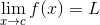Calculus 1‎ > ‎Calculus Lessons‎ > ‎Unit 1 - Limits‎ > ‎

### Day 02 - Finding Limits Graphically and Numerically - 01.05.16

 Update Unit 1 Test on 1/22ReviewLimitsWhat does “lim” stand for in the notation above?limlimitlimericknone of the aboveWhat does f(x) stand for in the notation above?an equation you are finding the limit ofa functionthe limitnone of the aboveWhat does x → c stand for?It represents the value of x getting infinitesimally closer to c, but never actually reaching it.It represent x being set equal to c.It represent x turning into c.none of the aboveWhat does the L stand for?It represents the value that the function is limited to.It represents the limit of the function.It represents the value of f(x) if x was getting infinitesimally closer to c.none of the aboveFind the f(1) for the following: undefined120none of the aboveLessonCalculus Challenge 1AWhat if we could find f(1) for ?What would it equal?Graph of function: https://www.desmos.com/calculator/qk9k8e6mdbCalculus Challenge 1BEvaluate the following limit:Graph of function: https://www.desmos.com/calculator/sbtshxk1wcLimitsHow are limits found numerically and graphically? (video) (checkpoints) Posted on the board at the end of the block.HomeworkStudy! Help Request List Standard(s) APC.2Define and apply the properties of limits of functions.Limits will be evaluated graphically and algebraically.Includes:​limits of a constant​limits of a sum, product, and quotient​one-sided limits​limits at infinity, infinite limits, and non-existent limits